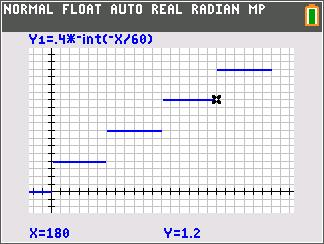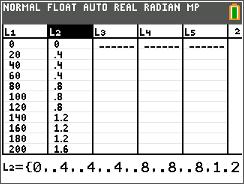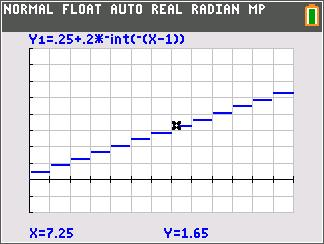••• ##### Device
• TI-84 Plus
• TI-84 Plus Silver Edition
•TI-84 Plus C Silver Edition
•TI-84 Plus CE
• ##### Report an Issue

Algebra I: Cell Phone Range
by Texas Instruments#### Overview

Students will learn to identify the domain and range of various real-world step functions. They will graphically explore numerical data points and observe step functions.

#### Key Steps

•Students are introduced to the step function. They will use lists to calculate the cost of a cell phone plan for minute usage. After each number is calculated, they will graph it as a scatter plot.

•Students will then view a graph of the step function and use the Trace tool to determine the range of the function. A discussion should follow about the difference between an open circle and closed circle.

•In the second problem, students will determine the domain and range of a cost function. They will use the graph to calculate the cost to mail a 7.25 ounce letter. There are two extension or homework problems that have students identify the domain and range of several different functions.